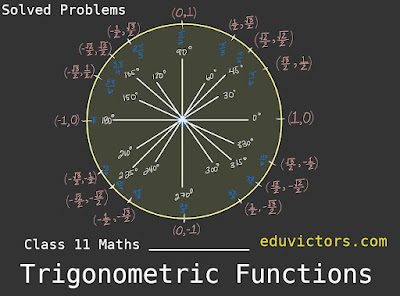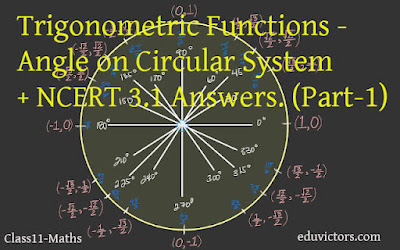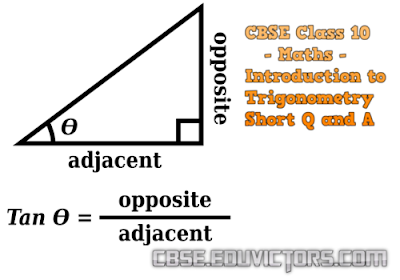## Pages

Showing posts with label Trigonometry. Show all posts
Showing posts with label Trigonometry. Show all posts

# Class 11 - Maths - Trigonometric Functions Notes, Solved Problems and NCERT Exercise 3.2 Solutions

Answer: All angles which are integral multiples of π/2 are called quadrantal angles.

Therefore, for quadrantal angles, we have# Class 11 - Maths - Trigonometric Functions - Angle on Circular System + NCERT 3.1 Answers. (Part-1)

The phrase "measuring the sides of a triangle" is how the word "trigonometry" is obtained from the Greek words "trigon" and "metron." The field was initially created to address triangle-related geometric issues. The surveyors who mapped out the new territories, the engineers, and other people studied it for navigation. Currently, numerous fields, including the science of seismology, the design of electrical circuits, the description of the condition of an atom, forecasting ocean tide heights, analysing musical tones, and many other things.

You should remember

1. In the sexagesimal system, we measure angles in degrees, minutes and seconds.

1 right angle = 90°

1° = 60′  (minute) and

1′ = 60′′ (second)

2. In the circular measure, we measure angles in radians.

Thus,

πc = 180°## Introduction to Trigonometry

Short Q and A

Q1: If sin 3A = cos (A – 26°), where 3A is an acute angle, find the value of A.

Answer: sin 3A = cos (A – 26°)

∵ sin 3A = cos (90° – 3A)
∴ cos (90° – 3A) = cos (A – 26°)

Since
90° – 3A and A – 26° are both acute angles, therefore,
90° – 3A = A – 26°
A = 29°

Q2: Express cot 85° + cos 75° in terms of trigonometric ratios of angles between 0° and 45°.

Answer: cot 85° + cos 75° = cot (90° – 5°) + cos (90° – 15°)

= tan 5° + sin 15°